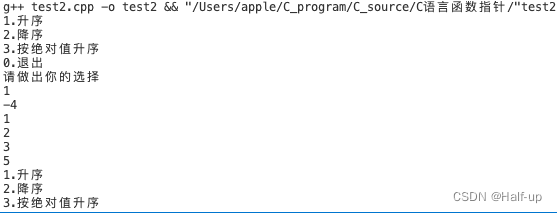• 函数指针的声明和使用
• 通过函数指针调用函数
• 函数指针做参数
• 函数指针数组

# 函数指针的声明和使用

`返回值类型 (*函数指针名)(参数列表);`

• `*函数指针名 `表示函数指针的名称
• `返回值类型` 则表示该指针所指向的函数的返回值类型
• `参数列表` 则表示该指针所指向的函数的参数列表。

``````int func(int x,int y); //声明一个函数
fp=func;//将函数func的首地址给fp指针
int (*fp)(int, int); //声明一个函数指针
``````

• 举例：func加法
``````// 声明一个函数
int func(int x, int y) {
return x + y;
}

int main() {
// 声明一个函数指针
int (*fp)(int, int);

// 将函数的首地址赋给函数指针
fp = func;

// 通过函数指针调用函数
int result = (*fp)(1, 2);    // result = 3

return 0;
}
``````

# 通过函数指针调用函数

``````// 声明一个函数
int func(int x, int y) {
return x + y;
}

int main() {
// 声明一个函数指针
int (*fp)(int, int);

// 将函数的首地址赋给函数指针
fp = func;

// 通过函数指针调用函数
int result = (*fp)(1, 2);    // result = 3

return 0;
}
``````

``````  // 通过函数指针调用函数
int result = (*fp)(1, 2);    // result = 3
``````

# 函数指针做参数

• 用函数指针实现带有菜单驱动的数组排序

1. 根据三种排序要求，分别定义三个排序函数
``````int asc(int x,int y);//升序排序，若x>y,则视为逆序返回1
int desc(int x,int y);//降序排序,若x<y,则视为逆序返回1
int AscAbs(int x,int y);//绝对值升序排序,若fabs(x)>fabs(y)则视为逆序返回1
``````
1. 为Sort函数添加一个指向函数的指针作为形参，sort()函数原型如下：
``````void Sort(int arr[],int n,int(*f)(int x,int y));
``````

1. 在调用sort函数时，根据不同的排序要求，选择不同的比较函数作为实参，例如下面：
``````Sort(a,n,asc);
``````

``````#include<stdio.h>
#include<math.h>

int asc(int x,int y);//升序排序，若x>y,则视为逆序返回1
int desc(int x,int y);//降序排序,若x<y,则视为逆序返回1
int AscAbs(int x,int y);//绝对值升序排序,若fabs(x)>fabs(y)则视为逆序返回1
void Sort(int arr[],int n,int(*f)(int x,int y));

int main()
{
int a={3,-4,1,5,2};
int select;
int n=5,i;

//菜单
while (1)
{
printf("1.升序\n");
printf("2.降序\n");
printf("3.按绝对值升序\n");
printf("0.退出\n");
printf("请做出你的选择\n");
scanf("%d",&select);

if(select==0)
break;

if(select<0&&select>3)
printf("请输入正确的选项\n");

//根据用户的选择，调用不同的比较函数
switch (select)
{
case 1: Sort(a,n,asc);break;
case 2: Sort(a,n,desc);break;
case 3: Sort(a,n,AscAbs);break;
default:
break;
}
for ( i = 0; i < 5; i++)
{
printf("%d ",a[i]);
printf("\n");
}
}
return 0;

}

int asc(int x,int y)
{
if(x>y)
return 1;
else
return 0;
}

int desc(int x,int y)
{
if(x<y)
return 1;
else
return 0;
}

int AscAbs(int x,int y)
{
if(fabs(x)>fabs(y))
return 1;
else
return 0;
}

void Sort(int arr[],int n,int(*f)(int x,int y))
{
int i,j,t;

//冒泡排序
for(i=0;i<n-1;i++)
{
for(j=0;j<n-i-1;j++)
{
if((*f)(arr[j],arr[j+1]))//逆序返回1
{
t=arr[j];
arr[j]=arr[j+1];
arr[j+1]=t;
}
}
}
}
``````# 函数指针数组

• 格式：`返回类型 (*指针数组名[数组长度])(形参列表);`

• 改进上面那个程序，变为函数指针数组形式
``````#include<stdio.h>
#include<math.h>

int asc(int x, int y);//升序排序，若x>y,则视为逆序返回1
int desc(int x, int y);//降序排序,若x<y,则视为逆序返回1
int AscAbs(int x, int y);//绝对值升序排序,若fabs(x)>fabs(y)则视为逆序返回1
void Sort(int arr[], int n, int(*f)(int x, int y));

int main()
{
int (*f[])(int x, int y) = {asc, desc, AscAbs};
int a = {3, -4, 1, 5, 2};
int select;
int n = 5, i;

//菜单
while (1)
{
printf("1.升序\n");
printf("2.降序\n");
printf("3.按绝对值升序\n");
printf("0.退出\n");
printf("请做出你的选择\n");
scanf("%d", &select);

if (select == 0)
break;

if (select < 0 || select > 3)
{
printf("请输入正确的选项\n");
continue;
}

printf("排序前: ");
for (i = 0; i < n; i++)
{
printf("%d ", a[i]);
}
printf("\n");

Sort(a, n, f[select - 1]);

printf("排序后: ");
for (i = 0; i < n; i++)
{
printf("%d ", a[i]);
}
printf("\n");
}
return 0;
}

int asc(int x, int y)
{
if (x > y)
return 1;
else
return 0;
}

int desc(int x, int y)
{
if (x < y)
return 1;
else
return 0;
}

int AscAbs(int x, int y)
{
if (fabs(x) > fabs(y))
return 1;
else
return 0;
}

void Sort(int arr[], int n, int(*f)(int x, int y))
{
int i, j, t;

//冒泡排序
for (i = 0; i < n - 1; i++)
{
for (j = 0; j < n - i - 1; j++)
{
if ((*f)(arr[j], arr[j + 1]))//逆序返回1
{
t = arr[j];
arr[j] = arr[j + 1];
arr[j + 1] = t;
}
}
}
}
``````